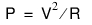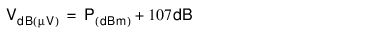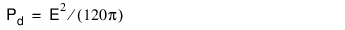MA2700A InterferenceHunter Help : Spectrum Analyzer : Field Strength : Antenna Calculations

Antenna Calculations
The following is a list of various antenna calculations should you find it necessary to convert from one parameter to another:
Conversion of signal levels from watts to volts in a 50 ohm system:where:
P = power in Watts , V = voltage level in Volts, and R = resistance in ohms
Note that 1mW = 10–3 W and 1µV = 10–6 V.
For power in dBm, and voltage in dB (µV):Power density to field strength:
An alternate measure of field strength is power density:where:
E is the field strength in V/m and Pd is the Power density in W/m2
Power density at a point:where:
Pd = power density in W/m
Pt = power transmitted in Watts
Gt = gain of transmitting antenna
r = distance from the antenna in meters
This equation is only valid in the far field, where electric and magnetic fields are related by the characteristic impedance of free space.